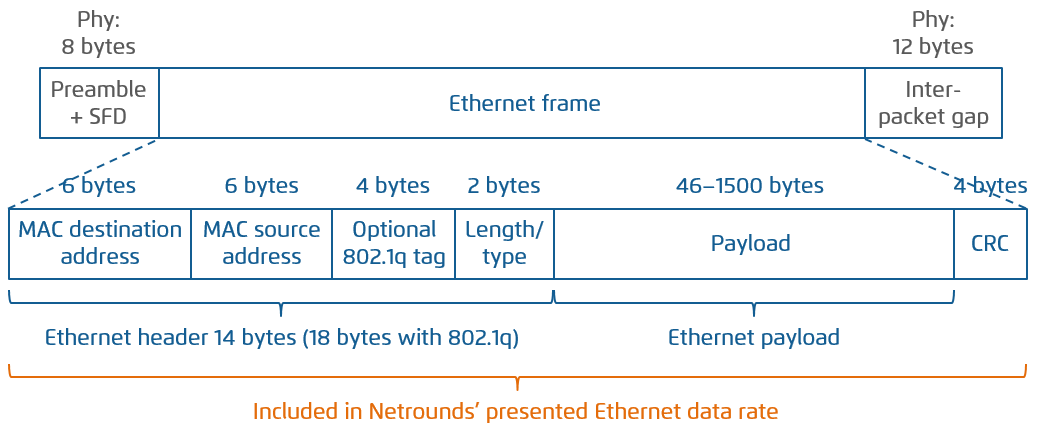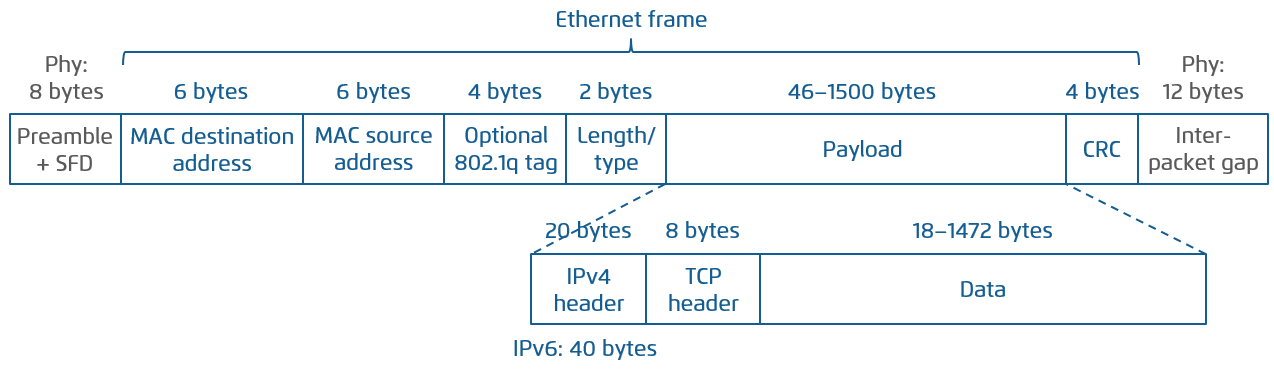# Theoretical maximum throughput with Netrounds Test Agents¶

This article discusses theoretical maximum throughput in Netrounds as compared to line rate, differences being due to protocol overhead. We frame our theoretical reasoning in the context of Netrounds Test Agents and their expected maximum performance. Besides overhead, there are other limiting factors which are not considered here, such as CPU limitations and overbooking of network links.

Theoretical performance is calculated for Ethernet and for TCP and UDP over Ethernet. Read more about Ethernet frame sizes here.

## Ethernet data rates¶The following table shows theoretical maximum Ethernet data rates in Netrounds UDP performance tests as percentages of the line rate. In these calculations, the header and CRC are included in the data rate. This is also the basis for the absolute Ethernet data rates presented in Netrounds.

Protocol Ethernet overhead (bytes) Theor. max. data rate (%)
Ethernet, no 802.1q,
MTU = 1500 bytes
8 preamble + 14 header + 4 CRC
+ 12 gap = 38 bytes/packet
(1500 + 18) / (1500 + 38)
= 98.6996%
Ethernet, no 802.1q,
MTU = 494 bytes
8 preamble + 14 header + 4 CRC
+ 12 gap = 38 bytes/packet
(494 + 18) / (494 + 38)
= 96.2406%
Ethernet, no 802.1q,
MTU = 46 bytes
8 preamble + 14 header + 4 CRC
+ 12 gap = 38 bytes/packet
(46 + 18) / (46 + 38)
= 76.1905%
Ethernet with 802.1q,
MTU = 1500 bytes
8 preamble + 18 header + 4 CRC
+ 12 gap = 42 bytes/packet
(1500 + 22) / (1500 + 42)
= 98.7030%
Ethernet with 802.1q,
MTU = 494 bytes
8 preamble + 18 header + 4 CRC
+ 12 gap = 42 bytes/packet
(494 + 22) / (494 + 42)
= 95.5224%
Ethernet with 802.1q,
MTU = 46 bytes
8 preamble + 18 header + 4 CRC
+ 12 gap = 42 bytes/packet
(46 + 22) / (46 + 42)
= 72.7273%

## TCP over Ethernet data rates¶The following table shows theoretical maximum TCP data rates in Netrounds TCP performance tests as percentages of the line rate. In these calculations, only TCP data (“Data” in the above figure) counts as payload. This is also how absolute TCP data rates are presented in Netrounds.

It is assumed throughout that no header compression takes place.

Protocol TCP/IP overhead (bytes) Theor. max. data rate (%)
TCP over Ethernet,
no 802.1q, IPv4
20 IPv4 + 20 TCP + 12 timestamps
= 52 bytes/packet
(1500 – 52) / (1500 + 38)
= 94.1482%
TCP over Ethernet,
802.1q, IPv4
20 IPv4 + 20 TCP + 12 timestamps
= 52 bytes/packet
(1500 – 52) / (1500 + 42)
= 93.9040%
TCP over Ethernet,
no 802.1q, IPv6
40 IPv6 + 20 TCP + 12 timestamps
= 72 bytes/packet
(1500 – 72) / (1500 + 38)
= 92.8479%
TCP over Ethernet,
802.1q, IPv6
40 IPv6 + 20 TCP + 12 timestamps
= 72 bytes/packet
(1500 – 72) / (1500 + 42)
= 92.6070%

## UDP over Ethernet data rates¶The following table shows theoretical maximum data rates in UDP tests as percentages of the line rate. These percentages are provided as a reference; no such figures are presented in Netrounds. Rather, the data rate presented for UDP streams is the Ethernet data rate (see above).

Protocol UDP/IP overhead (bytes) Theor. max. data rate (%)
UDP over Ethernet,
no 802.1q, IPv4
20 IPv4 + 8 UDP
= 28 bytes/packet
(1500 – 28) / (1500 + 38)
= 95.7087%
UDP over Ethernet,
802.1q, IPv4
20 IPv4 + 8 UDP
= 28 bytes/packet
(1500 – 28) / (1500 + 42)
= 95.4604%
UDP over Ethernet,
no 802.1q, IPv6
40 IPv6 + 8 UDP
= 48 bytes/packet
(1500 – 48) / (1500 + 38)
= 94.4083%
UDP over Ethernet,
802.1q, IPv6
40 IPv6 + 8 UDP
= 48 bytes/packet
(1500 – 48) / (1500 + 42)
= 94.1634%